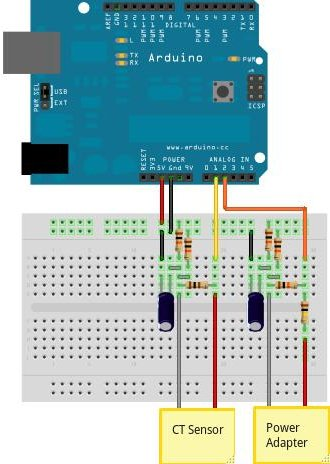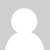## How to build an Arduino energy monitor - measuring mains voltage and current

Including voltage measurement via AC-AC voltage adapter and current measurement via a CT sensor.This guide details how to build a simple electricity energy monitor on that can be used to measure how much electrical energy you use in your home. It measures voltage with an AC to AC power adapter and current with a clip on CT sensor, making the setup quite safe as no high voltage work is needed.

The energy monitor can calculate real power, apparent power, power factor, rms voltage, rms current. All the calculations are done in the digital domain on an Arduino.

### Step One – Gather Components

You will need:

1x Arduino

Voltage sensing electronics:

1x 100kOhm resistor for step down voltage divider.

1x 10kOhm resistor for step down voltage divider.

2x 470kOhm (for voltage divider, any matching value resistor pair down to 10K)

1x 10uF capacitor

Current sensing electronics

1x CT sensor SCT-013-000

1x Burden resistor 18 Ohms if supply voltage is 3.3V or 33 Ohms if supply voltage is 5V.

2x 470kOhm (for voltage divider, any matching value resistor pair down to 10K)

1x 10uF capacitor

Other

1x A breadboard and some single core wire.

Oomlout do a good arduino + breadboard bundle here £29

### Step Two – Assemble the electronics

The electronics consist of the sensors (which produce signals proportional to the mains voltage and current) and the sensor electronics that convert these signals into a form the Arduino is happy with.

For a circuit diagram and detailed discussion of sensors and electronics see:

CT Senors - Introduction

CT Sensors - Interfacing with an Arduino

Measuring AC Voltage with an AC to AC power adapter

Assemble the components as in the diagram above.

### Step Three – Upload the Arduino Sketch

The Arduino sketch is the piece of software that runs on the Arduino. The Arduino converts the raw data from its analog input into a nice useful values and then outputs them to serial.

a) Download EmonLib from github and place in your arduino libraries folder.

b) Upload the voltage and current example:

```#include "EmonLib.h"             // Include Emon Library
EnergyMonitor emon1;             // Create an instance

void setup()
{
Serial.begin(9600);

emon1.voltage(2, 234.26, 1.7);  // Voltage: input pin, calibration, phase_shift
emon1.current(1, 111.1);       // Current: input pin, calibration.
}

void loop()
{
emon1.calcVI(20,2000);         // Calculate all. No.of wavelengths, time-out
emon1.serialprint();           // Print out all variables
}
```

c) Open the arduino serial window

You should now see a stream of values. These are from left to right: real power, apparent power, rms voltage, rms current and power factor.### Re: How to build an arduino energy monitor

If it helps anyone, I ordered my cts for SEEEDSTUDIO and I calculated my CT sensors to be 1200. I don't know if all of them are 1200 but if you are ordering yours from seeedstudio you might want to use 1200 instead of 1500.

If someone else has ordered cts from SEEEDSTUDIO and found that their cts sensors are different than 1200 please hit reply. I am just curious if 1200 turns is standard for them or if it varies.

Thanks.### Re: How to build an arduino energy monitor

i agree Step one – calibrating power factor..### Re: How to build an arduino energy monitor

I have downloaded the library and the sketch but i get a compile error.

maybe because i dont have the file ??
#include "WProgram.h"

I guess thats where all the calculations are done, cause i dont see them anymore on those two files.

Am I missing a file?

thanks

### Re: How to build an arduino energy monitor

Have you placed the library in the arduino libraries folder? The calculations are done in this library, declared on this line:

I think Wprogram is a core arduino library.### Re: How to build an arduino energy monitor

Ok on the wprogram.

but on the emon.h, where are the calculations? i only see variables defined.

old pde used to have all the calculations within 3000 number of samples, sumV, sumP etc### Re: How to build an arduino energy monitor

An array of photovoltaic cells can capture the sun’s rays and transform it into green electricity. Queensland’s climate is particularly suitable for solar energy generation, and can be used for household energy consumption.
If you are living in Queensland, there has never been a better time to convert your home’s conventional power supply into one that is run by solar power systems Citisolar Solar Power Queensland aims to help you gain the best rebate for quality products. You will need to do your research as not all solar panels are created equal and every company will give you a different rebate.

### Re: How to build an arduino energy monitor

The calculations are in emon.cpp, emon.h is the header file that declares variables and functions in emon.cpp.### Re: How to build an arduino energy monitor

I have put mainsACexample.pde and Emon.h in the same folder.
tried to compile...not working.

### Re: How to build an arduino energy monitor

Emon.h and Emon.cpp need to be placed in the arduino ide libraries folder, for example: arduino/libraries/Emon

Make sure you restart the arduino ide too as it needs to do this to recognise the library### Re: How to build an arduino energy monitor

In your arduino sketch LoggerSketch.pde available in the EnergyMonitor.tar.gz download you use a function call emonA.measure() which is resulting in an error can this be substituted by emonA.calculate() instaed?